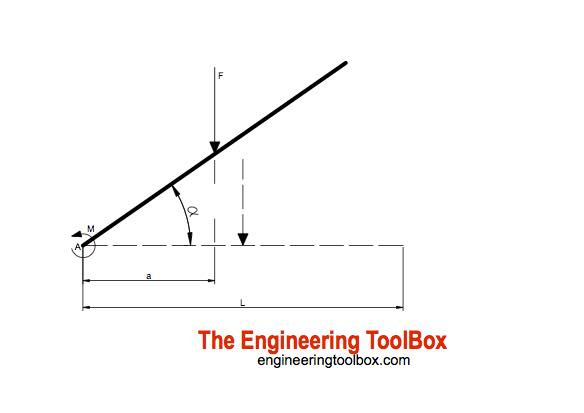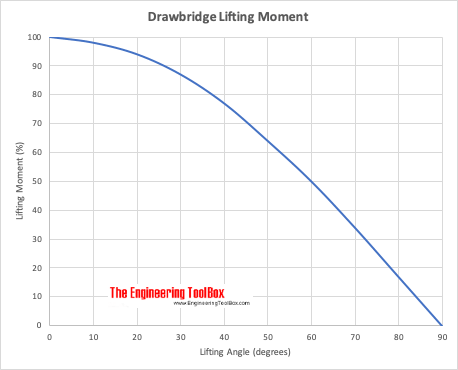Engineering ToolBox - Resources, Tools and Basic Information for Engineering and Design of Technical Applications!

# Drawbridge - Force and Moment vs. Elevation

## Calculate the acting forces and moments when elevating drawbridges or beams.

### Horizontal Beam/Drawbridge

For a beam supported at one end - like a typical drawbridge - the resultant force acting on the bridge can be calculated as

F = q L

= m g L              (1)

where

F = resultant force or weight (N)

q = uniform distributed load or weight (N/m)

L = length of beam or bridge (m)

m = continuous distributed mass (kg/m)

g = acceleration of gravity (9.81 m/s2)

For an uniform distributed load the resultant force F will act in distance

a = L / 2        (2)

The moment acting in A can be calculated as

M = F a              (3)

= F L / 2         (3b)

Note! The generic eq. 3 can be used for any combination of distributed or point loads.

#### Example - Moment with Horizontal Beam/Drawbridge

The total weight - or resultant force - of a 10 m HE-B 340B steel beam with continuous mass 134 kg/m can be calculated as

F = (134 kg/m) (9.81 m/s2) (10 m)

= 13145 N

The acting distance a for the resultant force or weight can be calculated as

a = (10 m) / 2

= 5 m

The moment acting in A can be calculated as

M = (13145 N) (5 m)

= 65725 Nm

###For and elevated beam or drawbridge the distance between the action force F and the moment in A varies. For a lifting angle the distance can be calculated as

a = cos(α) L / 2         (4)

where

α = lifting angle (degrees)

Eq. 3 for the moment can be modified to

M = F cos(α) L / 2          (5)

#### Example - Moment for Elevated Beam/Drawbridge

The beam from the example above is lifted 30 degrees. The moment in A can be calculated

M = (13145 N) cos(30 degrees) (10 m) / 2

= 56920 Nm

### Elevation Beam/Drawbridge Calculator

Default values are from the examples above where a = L / 2 in horizontal position. The calculator is generic and can be used for any uniform or point load combination as long as the resultant force F and point of action a can be calculated.

F - resultant force or weight  (N)

a - distance between resultant force and A in horizontal position (m)

#### Drawbridge Lifting Moment

Lifting moment (%) related to maximum start moment at elevation 0 degrees.## Related Topics

• Mechanics - Forces, acceleration, displacement, vectors, motion, momentum, energy of objects and more.
• Dynamics - Motion - velocity and acceleration, forces and torque.

## Engineering ToolBox - SketchUp Extension - Online 3D modeling!

Add standard and customized parametric components - like flange beams, lumbers, piping, stairs and more - to your Sketchup model with the Engineering ToolBox - SketchUp Extension - enabled for use with the amazing, fun and free SketchUp Make and SketchUp Pro .Add the Engineering ToolBox extension to your SketchUp from the SketchUp Pro Sketchup Extension Warehouse!

Translate

## Privacy

We don't collect information from our users. Only emails and answers are saved in our archive. Cookies are only used in the browser to improve user experience.

Some of our calculators and applications let you save application data to your local computer. These applications will - due to browser restrictions - send data between your browser and our server. We don't save this data.

## Citation

• Engineering ToolBox, (2006). Drawbridge - Force and Moment vs. Elevation . [online] Available at: https://www.engineeringtoolbox.com/moment-elevation-angle-beam-drawbridge-d_516.html [Accessed Day Mo. Year].

Modify access date.

. .

#### Scientific Online Calculator3 30